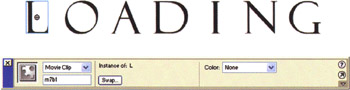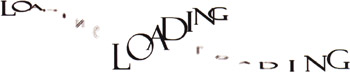In this example, we use a variation of the programmatic technique we developed in the last example to animate the word LOADING , for use in a preloader. Preloaders are small animations that are placed at the very beginning of a Flash movie and typically animate until the movie is completely loaded. If you aren't already familiar with preloaders and how to check if a movie is completely loaded, you can refer to any solid introductory Flash text. Also, you'll find some highly creative examples of preloaders in Flash MX Most Wanted: Effects and Movies ( friends of ED, ISBN:1-5959-224-7). To check out the finished version of this example, have a look at focus4.fla .

1. Press CTRL/CMD+N to create a new movie, and then set the document dimensions to 500x300 and the frame rate to 30 fps.

2. Use the Text Tool (T) to write the word LOADING in an attractive font. Select the LOADING text and then select Modify > Break Apart (CTRL/CMD+B) twice to convert the text into vector representations (once to break the word into individual characters , and then once again to break the letters down into vector graphics). Convert each character into a different movie clip by pressing F8. The symbol names are not important, but you do need to be able to control them from ActionScript, so the instance names are crucial. Use the Property Inspector (CTRL/CMD+F3) to name the letters in order: m1b1, m2b1, m3b1, m4b1, m5b1, m6b1, and m7b1 .3. In the previous example, you created a set_blur function that manipulated four copies of a sphere to make the sphere look fuzzy. Duplicating that code for each letter in the loading animation could be a lot of work, so you are going to move that functionality to the root movie. You can accomplish this by creating four copies of each letter. The three extra copies of letter m1b1 will be called m1b2, m1b3, and m1b4. The naming convention is actually very important because it lets you iterate over each unique movie clip and each duplicated movie clip.

Add the following ActionScript code to frame 1:

t = 0;  // The number of characters  n = 7;  // The number of character copies  b = 4;  // Create three additional copies of each character  depth = 100;   for (i=1; i<=n; i++) {   for (j=2; j<=b; j++) {   eval("m"+i+"b1").duplicateMovieClip("m"+i+"b"+j, depth);   depth++;   }   }   this.onEnterFrame = function() {  // increment the position along the path  t += .0582;  // Keep values in the range 0 to 2*pi  if (t>6.283) {   t -= 6.283;   }  // Iterate over all n (7) characters  for (i=  1  ; i<=n; i++) {  // offset each character by .2617 radians or 15 degrees  u = (t+i*.2617);  // v is used for scale as it corresponds to when u is       // farthest from the viewer  v = Math.abs(Math.cos(u/2));   c = Math.cos(u);  // dx, dy is the position of the character  dx = 50*c+35;   dy = 100*Math.sin(u)+150;  // Iterate over each copy of a particular character  for (j=1; j<=4; j++) {  // Reference a particular character copy  m = eval("m"+i+"b"+j);  // We offset each copy of the character and adjust         // the scale and alpha appropriately  offset = (1-v)*4;   m._yscale = c*100+100*v;   m._xscale = 200*v;   m._alpha = 10+90*v;   m._x = dx+offset*Math.cos(j*1.57)+50*(n+1-i);   m._y = dy+offset*Math.sin(j*1.57);   }   }   };
4. When you test this movie, CTRL/CMD+ENTER, you'll see that the letters really do seem to come forward from a fuzzy state to crisp clarity in this simple text effect.Flash 3D Cheats Most Wanted
ISBN: 1590592212
EAN: N/A
Year: 2002
Pages: 97